# How to add subtotals to a date field in excel in Excel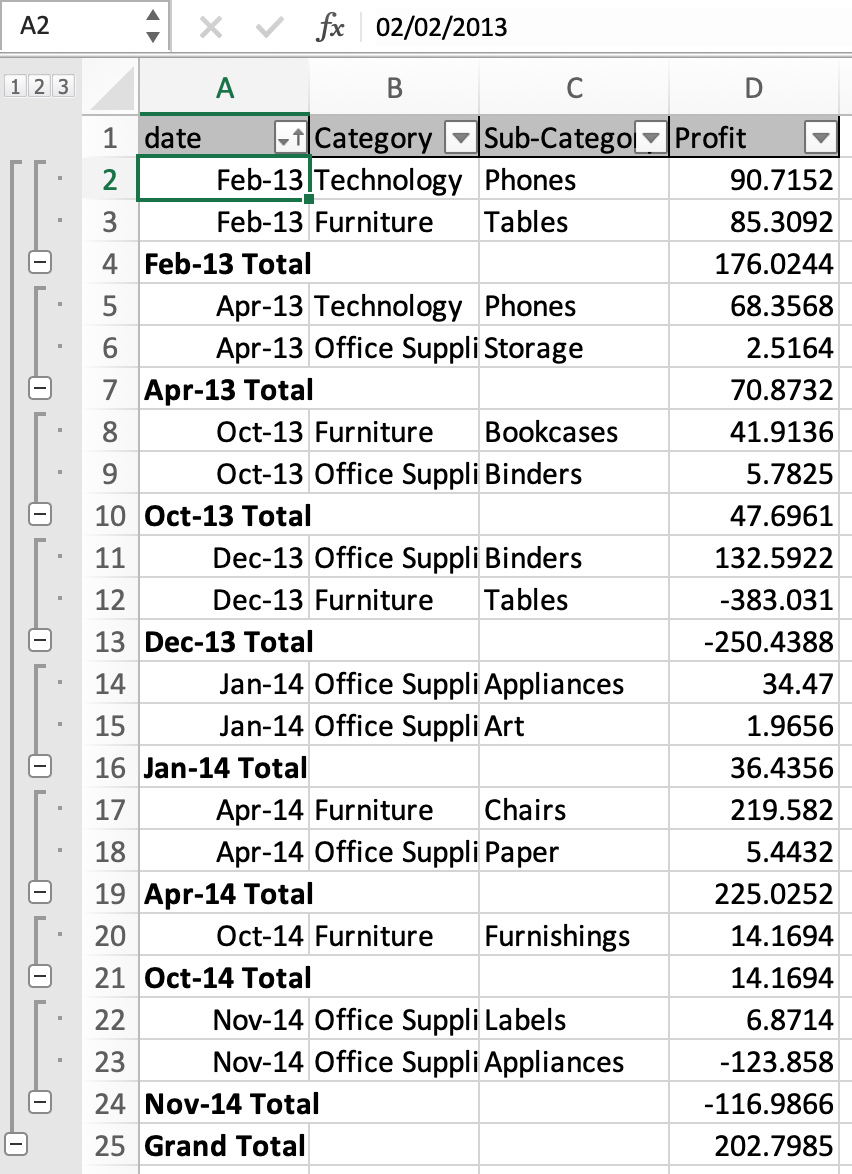In this article, we will learn How to add subtotals to a date field in excel in Excel.

Problem

Whenever a user accesses the subtotal option for the date column, it groups the data by each date value. But if we need to group data by month or year except by each date. For example sum employees tasks on the basis of month and year. For this we will use a feature of format cells and subtotal option to group data. Let's learn how it's done.

Format cells then Subtotal date field in Excel

Select the date range without column label. Right click > Format cells > Custom > choose mmm-yy > Click OK.

Go to Data > Subtotal > Select date column > Aggregate function > subtotal column > click OK.

Example :

All of these might be confusing to understand. Let's understand how to use the function using an example. Here we have a sample data.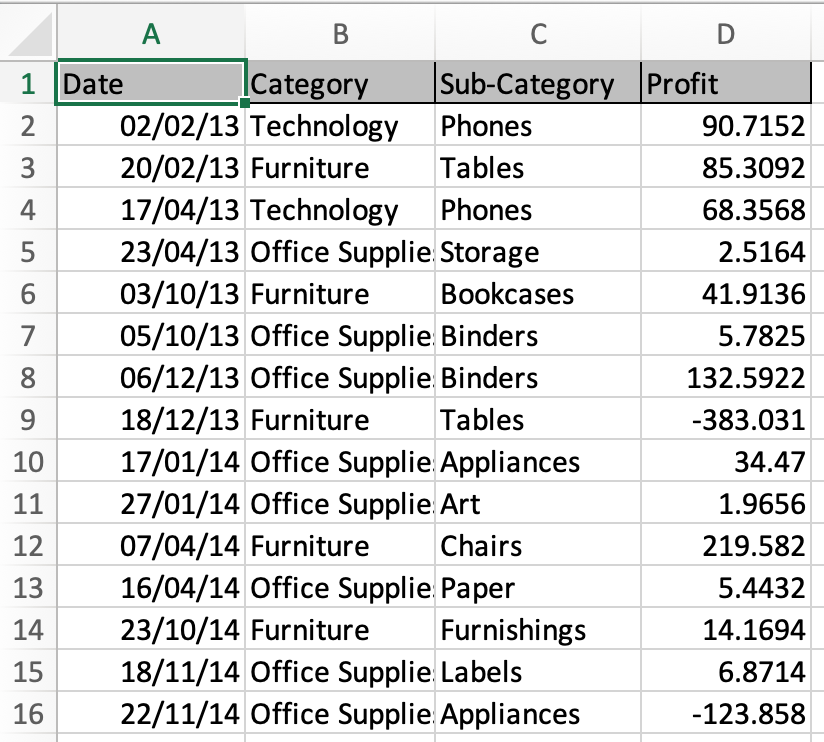Select the date range excluding the date column name and Right Click the range and Select Format cells option.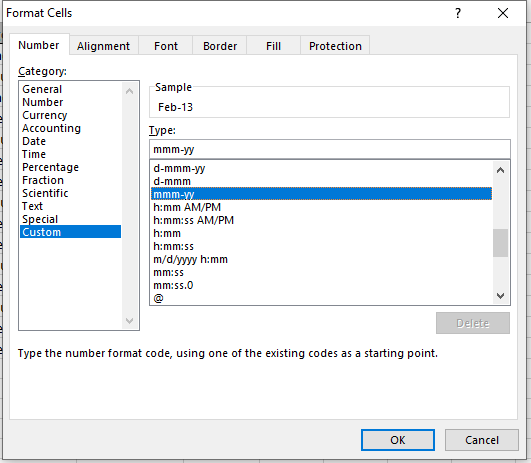A Format dialog box appears. Go the Custom option on the left pane and type mmm-yy and click Ok. Your date column is now in new format.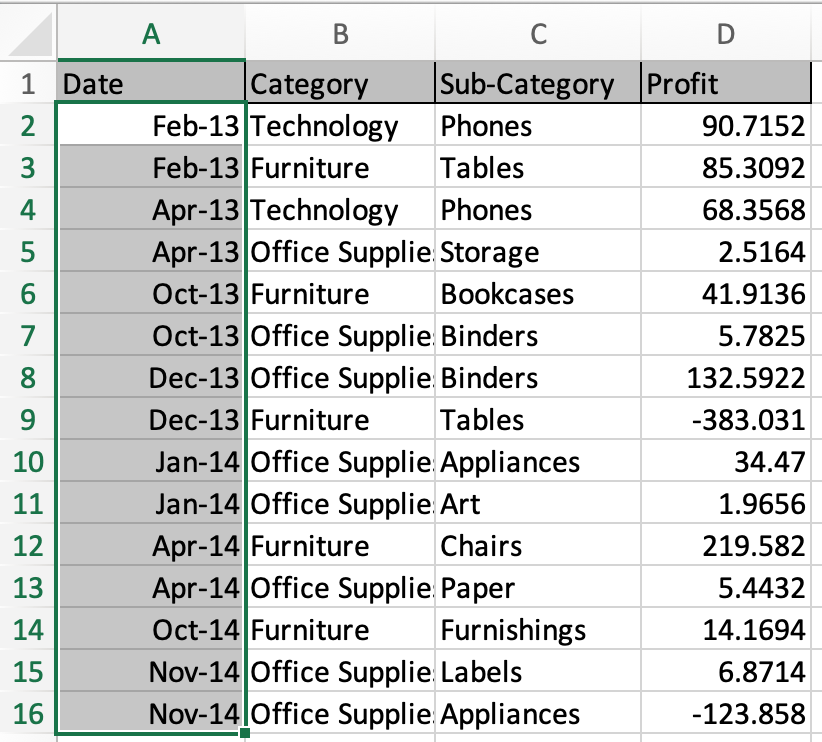As you can see your data is not edited. The cell still contains the date value. Now we proceed with the subtotaling date field. Select the table and Go to Data > Subtotal.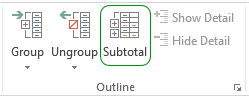Click Ok. And you can see the data is grouped as per new date format mmm-yy not the actual date value.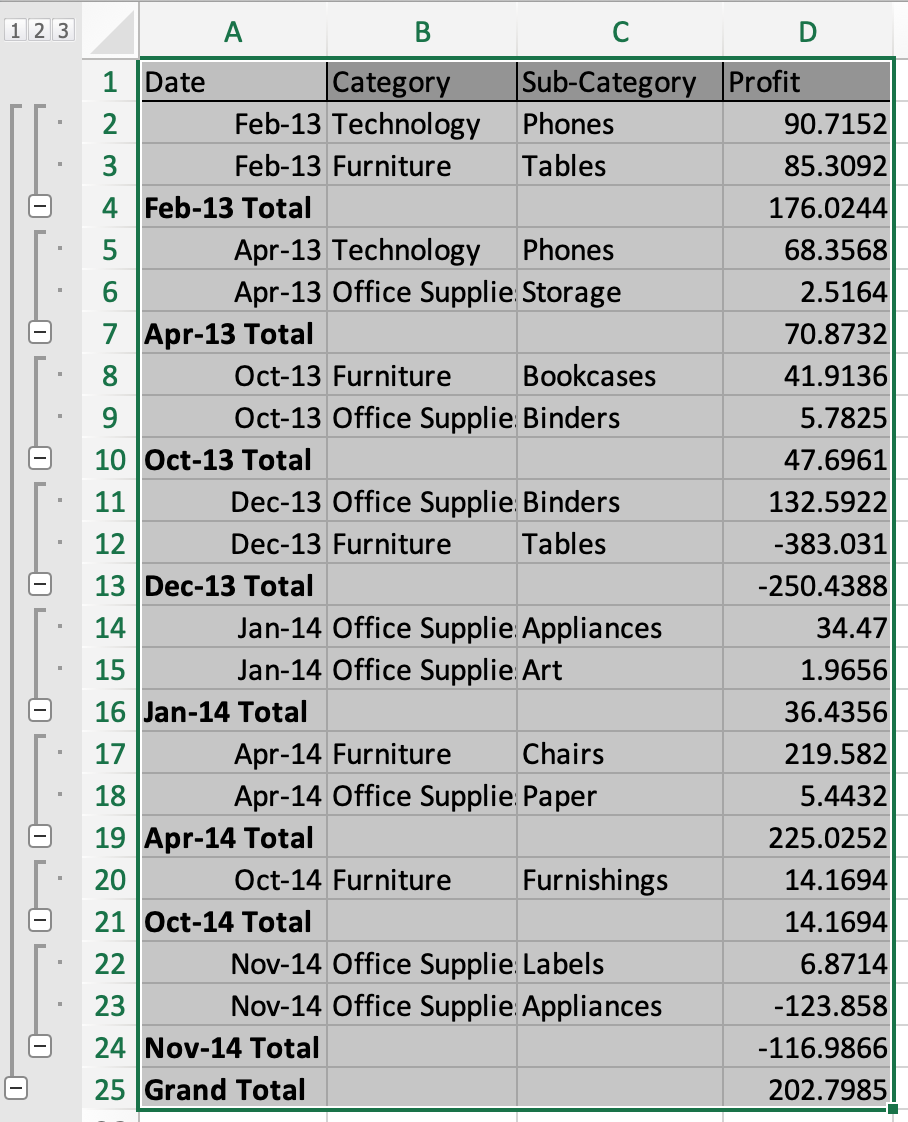Grouping date field values got a lot easier now. Isn't it?

Here are all the observational notes using the formula in Excel
Notes :

1. Select the data before proceeding to the subtotal option. Or else the function throws an error.
2. To remove subtotal. Select table and Go to Data > subtotal > click Remove all button (adjacent to Ok button).
3. Excel stores dates and time as numbers and is used in calculation by the function. So it’s recommended to use dates as cell reference or using the DATE function instead of giving direct argument to the function. Check the validation of date values, or else the function returns #VALUE! Error.

Hope this article about How to add subtotals to a date field in excel in Excel is explanatory. Find more articles on calculating values and related Excel formulas here. If you liked our blogs, share it with your friends on Facebook. And also you can follow us on Twitter and Facebook. We would love to hear from you, do let us know how we can improve, complement or innovate our work and make it better for you. Write to us at info@exceltip.com.

Related Articles :

How to use the SUBTOTAL function in Excel : Apply aggregate functions like average, sum, count, max, min on the grouped data using the subtotal function in Excel.

Count holidays between dates in Excel : count non working days between the two given dates using the DATEDIF and NETWORKDAYS function in Excel.

SUM price by weekdays in Excel : sum price values corresponding to the number of dates in a particular weekday using the SUMPRODUCT and WEEKDAY function in Excel.

Extract days between dates ignoring years in Excel : count the days between the two dates using different functions and mathematical operation in Excel

How to use the WORKDAYS function in Excel : Returns a workday date value after days added to the start_date using the WORKDAYS function in Excel.

Count Birth Dates By Month in Excel : count the number of dates lying in a particular month using the SUMPRODUCT and MONTH function in Excel.

Popular Articles :

How to use the IF Function in Excel : The IF statement in Excel checks the condition and returns a specific value if the condition is TRUE or returns another specific value if FALSE.

How to use the VLOOKUP Function in Excel : This is one of the most used and popular functions of excel that is used to lookup value from different ranges and sheets.

How to use the SUMIF Function in Excel : This is another dashboard essential function. This helps you sum up values on specific conditions.

How to use the COUNTIF Function in Excel : Count values with conditions using this amazing function. You don't need to filter your data to count specific values. Countif function is essential to prepare your dashboard.

Terms and Conditions of use

The applications/code on this site are distributed as is and without warranties or liability. In no event shall the owner of the copyrights, or the authors of the applications/code be liable for any loss of profit, any problems or any damage resulting from the use or evaluation of the applications/code.